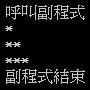1. 函數與副程式最基本的差異就是：函數有”回傳值”，副程式沒有。
2. 每一個函數或副程式只負責解決”一個”小問題。
3. 函數與副程式是程式裡的”黑盒子(Black Box)“，在黑盒子外面不需要知道裡面實作的方法，只需要知道輸入參數(Parameters)與回傳值。
4. 黑盒子出入口的介面(參數與回傳值)必須定義清楚並且容易了解。

VB.NET Sub副程式

Module Module1
Sub Main()  '程式執行的入口
Console.WriteLine("呼叫副程式")
printStar(1)
printStar(2)
printStar(3)
Console.WriteLine("副程式結束")

End Sub

'Sub 副程式名稱(傳遞方式 變數名稱 As 變數型態)
Sub printStar(ByVal n As Integer)   '輸出n個星星
If n <= 0 Then
Exit Sub
End If

For i As Integer = 1 To n
Label1.Text &= "*"
Next i
Label1.Text &= vbCrLf   '輸出換行符號vbCrLf
End Sub
End ModuleVB.NET Function函數

Module Module1
Sub Main()  '程式執行的入口
Dim a As Double = 3
Dim b As Double = 4
Dim c As Double = trigonometric(a, b)
Console.WriteLine("直角三角型二邊長為 " & a & " 和 " & b & "，則第三邊長為 " & c)

End Sub

'Function 函數名稱(傳遞方式 變數名稱 As 變數型態) As 回傳型態
Function trigonometric(ByVal a As Double, ByVal b As Double) As Double  '輸入直角三角型二個短邊長
Dim cSquare As Double = a ^ 2 + b ^ 2   '二邊長的平方相加等於鈄邊平方

'Return cSquare ^ 0.5
trigonometric = cSquare ^ 0.5   '回傳鈄邊長
Exit Function
End Function
End Module


Java Method方法

Java是物件導向程式語言，所以它用「方法(Method)」來稱呼函數，因為它的概念和傳統函數不太一樣，這在後面物件導向的章節中會加以說明。Java是屬於C風格程式語言，所以它的函數與副程式語法一樣，差別只在於有沒有回傳值。下面用一個完整範例來說明Java方法的使用方式，細部的解說請參考註解：

public class test
{
//main方法是程式的入口，執行程式從這裡開始
public static void main(String[] args)
{
System.out.println("呼叫副程式");
printStar(1);
printStar(2);
printStar(3);
System.out.println("副程式結束");
System.out.println();   //斷行

double a = 3;
double b = 4;
double c = trigonometric(a, b);
System.out.println("直角三角型二邊長為 " + a + " 和 " + b + "，則第三邊長為 " + c);
}

//方法基本宣告方式：
//物件導向的修飾字 回傳值 方法名稱(傳入參數) {}

//void表示沒有回傳值
private static void printStar(int n) {  //輸出n個星星
if(n != 0) {
for (int i = 0; i<n; i++) {
System.out.print("*");
}
System.out.println();
}
}

private static double trigonometric(double a, double b) {   //輸入直角三角型二個短邊長
//Math.pow(底數, 次方)：Java計算次方的方法，回傳double值
double cSquare = Math.pow(a, 2) + Math.pow(b, 2);   //二邊長的平方相加等於鈄邊平方

return Math.pow(cSquare, 0.5);  //回傳鈄邊長
}
}


double trigonometric(double a, double b) {}